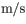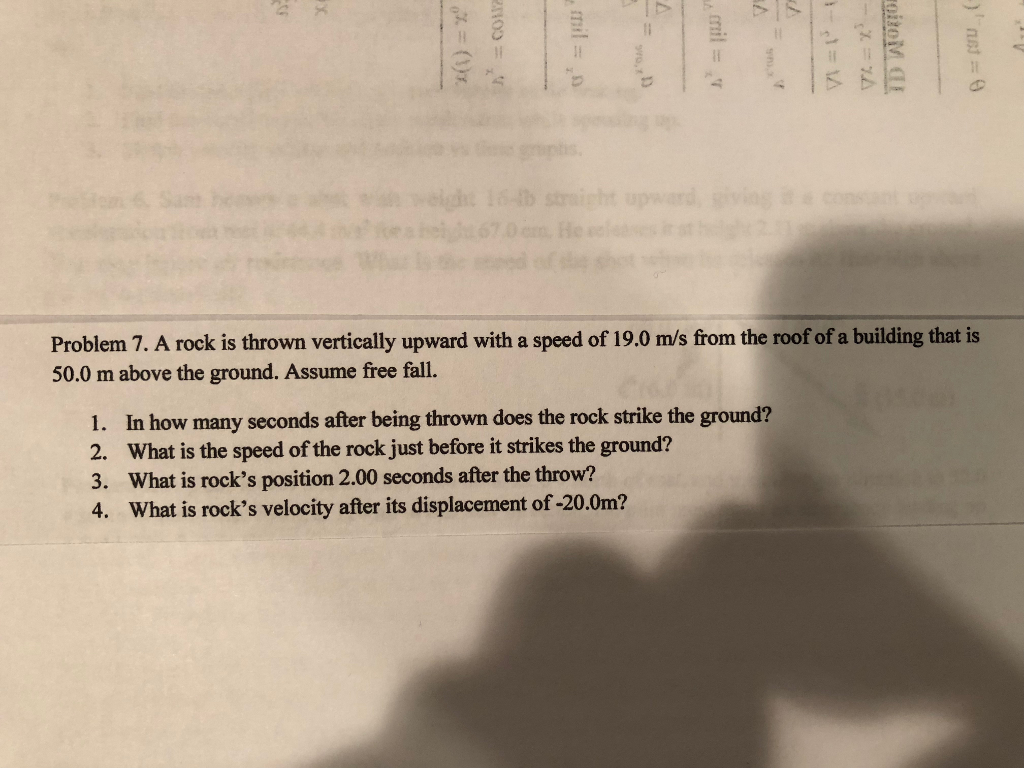# In this problem, you will apply kinematic equations to a jumping flea.

problem 1)

In this problem, you will apply kinematic equations to a jumping flea. Take the magnitude of free-fall acceleration to be 9.80m/s2 . Ignore air resistance.

1. A flea jumps straight up to a maximum height of 0.510m. What is its initial velocity as it leaves the ground?
3.16m/s

2. How long is the flea in the air from the time it jumps to the time it hits the ground?
time in air=____s

Problem 2)

A rock is thrown vertically upward with a speed of 19.0from the roof of a building that is 50.0 m above the ground. Assume free fall.1. In how many seconds after being thrown does the rock strike the ground?t=___s2.

What is the speed of the rock just before it strikes the ground?

Concept and reason

Use the concept of equations of motion, which includes the initial speed, final speed, acceleration due to gravity, time, and displacement covered to solve this problem.

Use the formula for maximum height reached to calculate the initial velocity. Then, use the formula for time of flight to calculate the time of flight. Use the equation of motion that relates displacement, acceleration, and time to calculate the time. Then use the equation of motion that relates initial and final velocities and time to calculate the velocity of the rock on reaching the ground.

Fundamentals

Displacement is defined as the smallest distance between two points.

Velocity is defined as the rate of change of displacement.

Acceleration is defined as the rate of change of velocity.

The relation between the initial velocity, final velocity, acceleration, and the displacement covered is,

${v^2} - {u^2} = 2as$

Here, u is the initial velocity, v is the final velocity, a is the acceleration, and s is the displacement.

The displacement is given by,

$s = ut + \frac{1}{2}a{t^2}$

Here, u is the initial velocity, a is the acceleration, and t is the time.

The velocity of the rock on reaching the ground is,

$v = u - gt$

Here, u is the initial velocity, g is the acceleration due to gravity, and t is the time.

The maximum height reached by an object projected vertically upwards is,

$h = \frac{{{u^2}}}{{2g}}$

Here, u is the initial velocity and g is the acceleration due to gravity.

The total time for which the object remains in air is called time of flight.

The total time of flight is given by,

$T = \frac{{2u}}{g}$

Here, u is the initial velocity and g is the acceleration due to gravity.

(1-1)

The maximum height reached by the flea is,

$h = \frac{{{u^2}}}{{2g}}$

Rearrange the equation for the initial velocity.

$u = \sqrt {2gh}$

Substitute $9.80{\rm{ m/}}{{\rm{s}}^2}$ for g and $0.510{\rm{ m}}$ for h.

$\begin{array}{c}\\u = \sqrt {2\left( {9.80{\rm{ m/}}{{\rm{s}}^2}} \right)\left( {0.510{\rm{ m}}} \right)} \\\\ = 3.16{\rm{ m/s}}\\\end{array}$

(1-2)

The total time of flight of the flea is,

$T = \frac{{2u}}{g}$

Here, u is the initial velocity and g is the acceleration due to gravity.

Substitute $3.16{\rm{ m/s}}$ for u and $9.80{\rm{ m/}}{{\rm{s}}^2}$ for g.

$\begin{array}{c}\\T = \frac{{2\left( {3.16{\rm{ m/s}}} \right)}}{{9.80{\rm{ m/}}{{\rm{s}}^2}}}\\\\ = 0.645{\rm{ s}}\\\end{array}$

(2-1)

The displacement is given by,

$s = ut + \frac{1}{2}a{t^2}$

Here, u is the initial velocity, a is the acceleration, and t is the time.

The rock falls down so the displacement is negative. The acceleration of the rock is due to gravity.

Substitute $- h$ for s and $- g$ for a.

$\begin{array}{c}\\ - h = ut + \frac{1}{2}\left( { - g} \right){t^2}\\\\g{t^2} - 2ut - 2h = 0\\\end{array}$

Substitute $19.0{\rm{ m/s}}$ for u, $9.80{\rm{ m/}}{{\rm{s}}^2}$ for g, and $50.0{\rm{ m}}$ for h.

$\begin{array}{c}\\\left( {9.80{\rm{ m/}}{{\rm{s}}^2}} \right){t^2} - 2\left( {19.0{\rm{ m/s}}} \right)t - 2\left( {50.0{\rm{ m}}} \right) = 0\\\\t = 5.675{\rm{ s}}\\\end{array}$

Round off to three significant figures. So, the time taken by the rock to reach the ground is$5.68{\rm{ s}}$.

(2-2)

The velocity of the rock on reaching the ground is,

$v = u - gt$

Substitute $19.0{\rm{ m/s}}$ for u, $9.80{\rm{ m/}}{{\rm{s}}^2}$ for g, and $5.675{\rm{ s}}$ for t.

$\begin{array}{c}\\v = \left( {19.0{\rm{ m/s}}} \right) - \left( {9.80{\rm{ m/}}{{\rm{s}}^2}} \right)\left( {5.675{\rm{ s}}} \right)\\\\ = - 36.6{\rm{ m/s}}\\\end{array}$

The negative sign indicates that the rock is falling down. The magnitude of velocity of the rock on reaching the ground is$36.6{\rm{ m/s}}$.

Ans: Part 1-1

The velocity with which the flea leaves the ground is$3.16{\rm{ m/s}}$.

Part 1-2

The flea is in the air for$0.645{\rm{ s}}$.

Part 2-1

The time after which the rock reaches the ground is$5.68{\rm{ s}}$.

Part 2-2

The magnitude of velocity of the rock on reaching the ground is$36.6{\rm{ m/s}}$.

#### Earn Coin

Coins can be redeemed for fabulous gifts.

Similar Homework Help Questions
• ### In this problem, you will apply kinematic equations to a jumping flea. Take the magnitude of...

In this problem, you will apply kinematic equations to a jumping flea. Take the magnitude of free-fall acceleration to be 9.80 m/s2 . Ignore air resistance.A flea jumps straight up to a maximum height of 0.390 m . What is its initial velocity v0 as it leaves the ground?

• ### Problem 7. A rock is thrown vertically upward with a speed of 19.0 m/s from the...Problem 7. A rock is thrown vertically upward with a speed of 19.0 m/s from the roof of a building that is 50.0 m above the ground. Assume free fall. 1. In how many seconds after being thrown does the rock strike the ground? 2. What is the speed of the rock just before it strikes the ground? 3. What is rock's position 2.00 seconds after the throw? 4. What is rock's velocity after its displacement of -20.0m?

• ### Acceleration

In this problem, you will apply kinematic equations to a jumping flea. Take the magnitude of free-fall acceleration to be 9.80 m/s2 . Ignore air resistance.How long is the flea in the air from the time it jumps to the time it hits the ground?

• ### Freefall problems; dealing with the kinematic equations.

In this problem, you will apply kinematic equations to a jumping flea. Take the magnitude of free-fall acceleration to be 9.80 m/s^2. Ignore air resistance.A flea jumps straight up to a maximum height of 0.390 m. What is its initial velocity v_0 as it leaves the ground?-I used the hints application and it told me that I had acceleration and final velocity. I thought I had acceleration and delta X...? I just don't get how to calculatethis "final velocity" they...

• ### PHYSICS :(

In this problem, you will apply kinematic equations to a jumping flea. Take the magnitude of free-fall acceleration to be 9.80 . Ignore air resistance.A flea jumps straight up to a maximum height of 0.380 . What is its initial velocity as it leaves the ground?

• ### A rock is thrown upward with a speed of 17.0 m/s from the roof of a building that is 50.0 m...

A rock is thrown vertically upward with a speed of 17.0 m/s from the roof of a building that is 50.0 m above the ground. Assume free fall.In how many seconds after being thrown does the rock strike the ground?

• ### A rock is thrown vertically upward with a speed of 11.0 m/s from the roof of...

A rock is thrown vertically upward with a speed of 11.0 m/s from the roof of a building that is 60.0 mabove the ground. Assume free fall. 1) In how many seconds after being thrown does the rock strike the ground? 2)What is the speed of the rock just before it strikes the ground?

• ### Ch 24-27 HW Problem 2.54 Part A A rock is thrown vertically upward with a speed...Ch 24-27 HW Problem 2.54 Part A A rock is thrown vertically upward with a speed of 17.0 m/s from the roof of a building that is 60.0 m above the ground. Assume free fall. In how many seconds after being thrown does the rock strike the ground AEQ * R O = ? Submit Request Answer Part B What is the speed of the rock just before it strikes the ground? % AXO O ? m/s Uground Submit Request...

• ### speed

A rock is thrown vertically upward with a speed of 19.0m/s from the roof of a building that is 50.0m above the ground. Assume free fall.What is the speed of the rock just before it strikes the ground?

• ### A small rock is thrown vertically upward with a speed of 23.0 m/s from the edge...

A small rock is thrown vertically upward with a speed of 23.0 m/s from the edge of the roof of a 39.0-m-tall building. The rock doesn't hit the building on its way back down and lands in the street below. Ignore air resistance. What is the speed of the rock just before it hits the street? How much time elapses from when the rock is thrown until it hits the street?